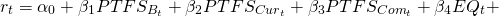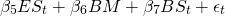# Fung-Hsieh 7 Factor Model

The Fung-Hsieh 7 factor model is a risk factor model commonly used to evaluate hedge funds’ performance. The seven factors are risk factors that explain a large proportion of the returns of hedge funds. The model was proposed door David Hsieh and William Fung in 2001 in a paper titled Hedge Fund Benchmarks: A Risk-Based Approach”. The aim of the factors is to capture the returns to a well-diversified portfolio of hedge funds.

On this page, we discuss the Fung-Hsieh 7 factor model definition. In particular, we discuss the risk factors that are included in the model. We also briefly introduce the Fung-Hsieh 8 factor model.

## Fung-Hsieh 7 factor model definition

The 7 factor model for hedge funds is actually a simple linear factor model, similar to the Fama and French 3 factor model for individual stocks. In this case, however, the model is used to explain hedge fund returns.

The model uses the following 7 factors:

• Bond Trend-Following Factor
• Currency Trend-Following Factor
• Commodity Trend-Following Factor
• Equity Market Factor
• The Equity Size Spread Factor
• The Bond Market Factor
• The Bond Size Spread Factor

The first three factors are trend-following factors proposed by Fung and Hsieh in a different paper called “The Risk in Hedge Fund Strategies: Theory and Evidence from Trend Followers“. These factors are available here.

Next, the equity market factor is captured using the S&P 500, the size factor is the difference between the Russell 2000 index monthly total return – Standard & Poor’s 500 monthly total return. The bond market factor is proxied using the the monthly change in the 10-year treasury constant maturity yield Finally, the size spread factor is measured using the monthly change in the Moody’s Baa yield less 10-year treasury constant maturity yield (month end-to-month end).

## Fung-Hsieh 7 factor model formula

Once we have the factors, the model looks as followswhere PTFS are the trend-following factors for Bonds, Currencies, and Commodities, EQ is the equity factor, ES is the Equity Size factor, BM is the bond market, and BS is the Bond Size factor.

## Fung-Hsieh 8 factor model

A few years after the introduction of the 7 factor model, Fung and Hsieh added an eighth factor. This model is referred to as the Fung-Hsieh 8-factor model. The additional factor that is added to the model is the MSCI Emerging Market index.

## Summary

We discussed the Fung-Hsieh 7 factor model. This is the workhorse model when researchers try to explain hedge funds’ return and analyze whether hedge funds generate alpha.